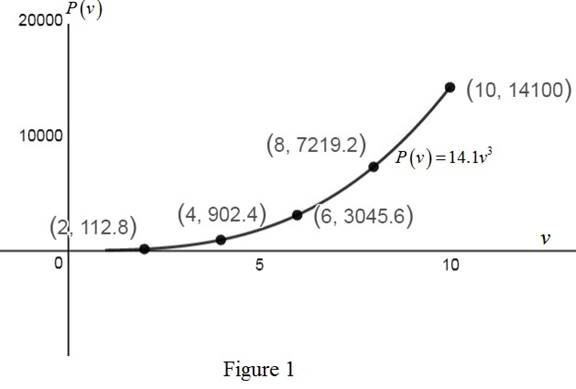# The graph for the given function P ( v ) = 14.1 v 3 .### Precalculus: Mathematics for Calcu...

6th Edition
Stewart + 5 others
Publisher: Cengage Learning
ISBN: 9780840068071### Precalculus: Mathematics for Calcu...

6th Edition
Stewart + 5 others
Publisher: Cengage Learning
ISBN: 9780840068071

#### Solutions

Chapter 2.2, Problem 80E
To determine

## To sketch: The graph for the given function P(v)=14.1v3.

Expert Solution

### Explanation of Solution

Given:

The length of the blade is 3 meters.

Power produced by the turbine is given by the function P(v)=14.1v3, where v is the speed of the wind.

Power measured in watts and velocity measured in meter per second.

Wind speed lies between 1m/sand10m/s.

Calculation:

Substitute different values for v and tabulate the corresponding values of P(v) as follows,

 v P(v) 2 P(2)=14.1(2)3=112.8 4 P(4)=14.1(4)3=4,902.4 6 P(6)=14.1(6)3=3,045.6 8 P(8)=14.1(8)3=7,219.2 10 P(10)=14.1(10)3=14,100

Sketch the graph of the function P(v)=14.1v3 as shown in the figure below.From Figure 1, it can be observed that the curve represents the graph of the power produced when the speed of the wind is in between 1 m/s and 10 m/s.

### Have a homework question?

Subscribe to bartleby learn! Ask subject matter experts 30 homework questions each month. Plus, you’ll have access to millions of step-by-step textbook answers!